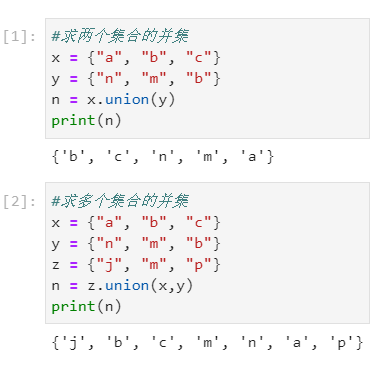### Python中求集合并集的方法union()方法

【小白从小学Python、C、Java】

【Python全国计算机等级考试】

【Python数据分析考试必会题】

● 标题与摘要

Python中求集合并集的方法

union()方法

● 选择题

x = {“a”, “b”, “c”}

y = {“n”, “m”, “b”}

n = x.union(y)

print(n)

A {‘c’, ‘m’, ‘b’, ‘n’, ‘a’}

B {‘c’, ‘m’, ‘b’, ‘n’, ‘a’, ‘b’}

C {‘c’, ‘m’, ‘b’, ‘n’, ‘b’}

D {‘c’, ‘m’, ‘b’, ‘n’, ‘a’, ‘m’}

● 问题解析

1.union()函数用于求集合的并集。并集是指给定多个集合，把他们所有的元素合并在一起组成的集合，新形成的集合称为这多个集合的并集。

2.union()方法语法：set0.union(set1，set2…),其中set1为必填集合，作为合并的目标集合，set2等集合是选填集合，作为其他要进行合并的集合，可以多个，多个使用逗号隔开。代码含义是：将set0、set1、set2等集合进行合并，集合中的重复元素只出现一次。

3.本题中首先定义了两个集合，分别为x = {“a”, “b”, “c”}、y = {“n”, “m”, “b”}。在进行x.union(y)时，x、y中共包括’a’, ‘b’, ‘c’, ‘n’, ‘m’这五个元素，因此求出的并集应同时包含这五个元素，同时由于集合中的元素不可重复，并集也是集合，所以并集中的元素没有重复项。综合以上因素，所求并集应同时包含’a’, ‘b’, ‘c’, ‘n’, ‘m’这五个元素，且无重复元素。

● 附图● 附图代码

#求两个集合的并集

x = {“a”, “b”, “c”}

y = {“n”, “m”, “b”}

n = x.union(y)

print(n)

#求多个集合的并集

x = {“a”, “b”, “c”}

y = {“n”, “m”, “b”}

z = {“j”, “m”, “p”}

n = z.union(x,y)

print(n)

● 正确答案

A

● 温馨期待

欢迎大家转发，一起传播知识和正能量，帮助到更多人。期待大家提出宝贵改进建议，互相交流，收获更大。辛苦大家转发时注明出处(也是咱们公益编程交流群的入口网址)，刘经纬老师共享知识相关文件下载地址为：http://liujingwei.cn